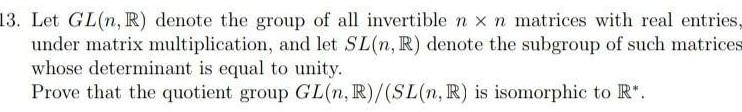Question:

# 13 Let GL n R denote the group of all invertible n x n

Last updated: 9/13/202313 Let GL n R denote the group of all invertible n x n matrices with real entries under matrix multiplication and let SL n R denote the subgroup of such matrices whose determinant is equal to unity Prove that the quotient group GL n R SL n R is isomorphic to R